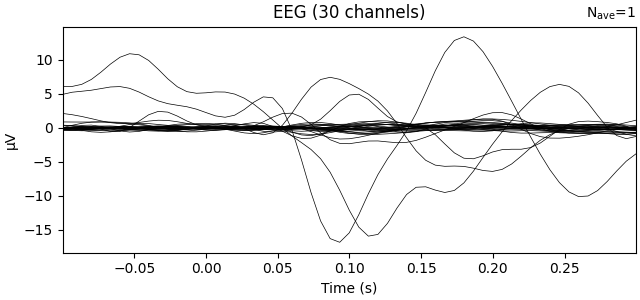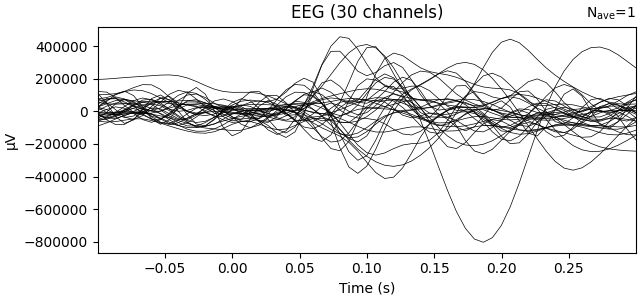# Analysis of evoked response using ICA and PCA reduction techniques#

This example computes PCA and ICA of evoked or epochs data. Then the PCA / ICA components, a.k.a. spatial filters, are used to transform the channel data to new sources / virtual channels. The output is visualized on the average of all the epochs.

```# Authors: Jean-Remi King <jeanremi.king@gmail.com>
#          Asish Panda <asishrocks95@gmail.com>
#
```
```import numpy as np
import matplotlib.pyplot as plt

import mne
from mne.datasets import sample
from mne.decoding import UnsupervisedSpatialFilter

from sklearn.decomposition import PCA, FastICA

print(__doc__)

# Preprocess data
data_path = sample.data_path()

# Load and filter data, set up epochs
meg_path = data_path / 'MEG' / 'sample'
raw_fname = meg_path / 'sample_audvis_filt-0-40_raw.fif'
event_fname = meg_path / 'sample_audvis_filt-0-40_raw-eve.fif'
tmin, tmax = -0.1, 0.3
event_id = dict(aud_l=1, aud_r=2, vis_l=3, vis_r=4)

raw.filter(1, 20, fir_design='firwin')

picks = mne.pick_types(raw.info, meg=False, eeg=True, stim=False, eog=False,

epochs = mne.Epochs(raw, events, event_id, tmin, tmax, proj=False,
verbose=False)

X = epochs.get_data()
```
```Opening raw data file /home/circleci/mne_data/MNE-sample-data/MEG/sample/sample_audvis_filt-0-40_raw.fif...
Read a total of 4 projection items:
PCA-v1 (1 x 102)  idle
PCA-v2 (1 x 102)  idle
PCA-v3 (1 x 102)  idle
Average EEG reference (1 x 60)  idle
Range : 6450 ... 48149 =     42.956 ...   320.665 secs
Reading 0 ... 41699  =      0.000 ...   277.709 secs...
Filtering raw data in 1 contiguous segment
Setting up band-pass filter from 1 - 20 Hz

FIR filter parameters
---------------------
Designing a one-pass, zero-phase, non-causal bandpass filter:
- Windowed time-domain design (firwin) method
- Hamming window with 0.0194 passband ripple and 53 dB stopband attenuation
- Lower passband edge: 1.00
- Lower transition bandwidth: 1.00 Hz (-6 dB cutoff frequency: 0.50 Hz)
- Upper passband edge: 20.00 Hz
- Upper transition bandwidth: 5.00 Hz (-6 dB cutoff frequency: 22.50 Hz)
- Filter length: 497 samples (3.310 sec)

[Parallel(n_jobs=1)]: Using backend SequentialBackend with 1 concurrent workers.
[Parallel(n_jobs=1)]: Done   1 out of   1 | elapsed:    0.0s remaining:    0.0s
[Parallel(n_jobs=1)]: Done   2 out of   2 | elapsed:    0.0s remaining:    0.0s
[Parallel(n_jobs=1)]: Done   3 out of   3 | elapsed:    0.0s remaining:    0.0s
[Parallel(n_jobs=1)]: Done   4 out of   4 | elapsed:    0.0s remaining:    0.0s
[Parallel(n_jobs=1)]: Done 366 out of 366 | elapsed:    0.6s finished
```

Transform data with PCA computed on the average ie evoked response

```pca = UnsupervisedSpatialFilter(PCA(30), average=False)
pca_data = pca.fit_transform(X)
ev = mne.EvokedArray(np.mean(pca_data, axis=0),
mne.create_info(30, epochs.info['sfreq'],
ch_types='eeg'), tmin=tmin)
ev.plot(show=False, window_title="PCA", time_unit='s')
```Transform data with ICA computed on the raw epochs (no averaging)

```ica = UnsupervisedSpatialFilter(
FastICA(30, whiten='unit-variance'), average=False)
ica_data = ica.fit_transform(X)
ev1 = mne.EvokedArray(np.mean(ica_data, axis=0),
mne.create_info(30, epochs.info['sfreq'],
ch_types='eeg'), tmin=tmin)
ev1.plot(show=False, window_title='ICA', time_unit='s')

plt.show()
```Total running time of the script: ( 0 minutes 5.457 seconds)

Estimated memory usage: 128 MB

Gallery generated by Sphinx-Gallery